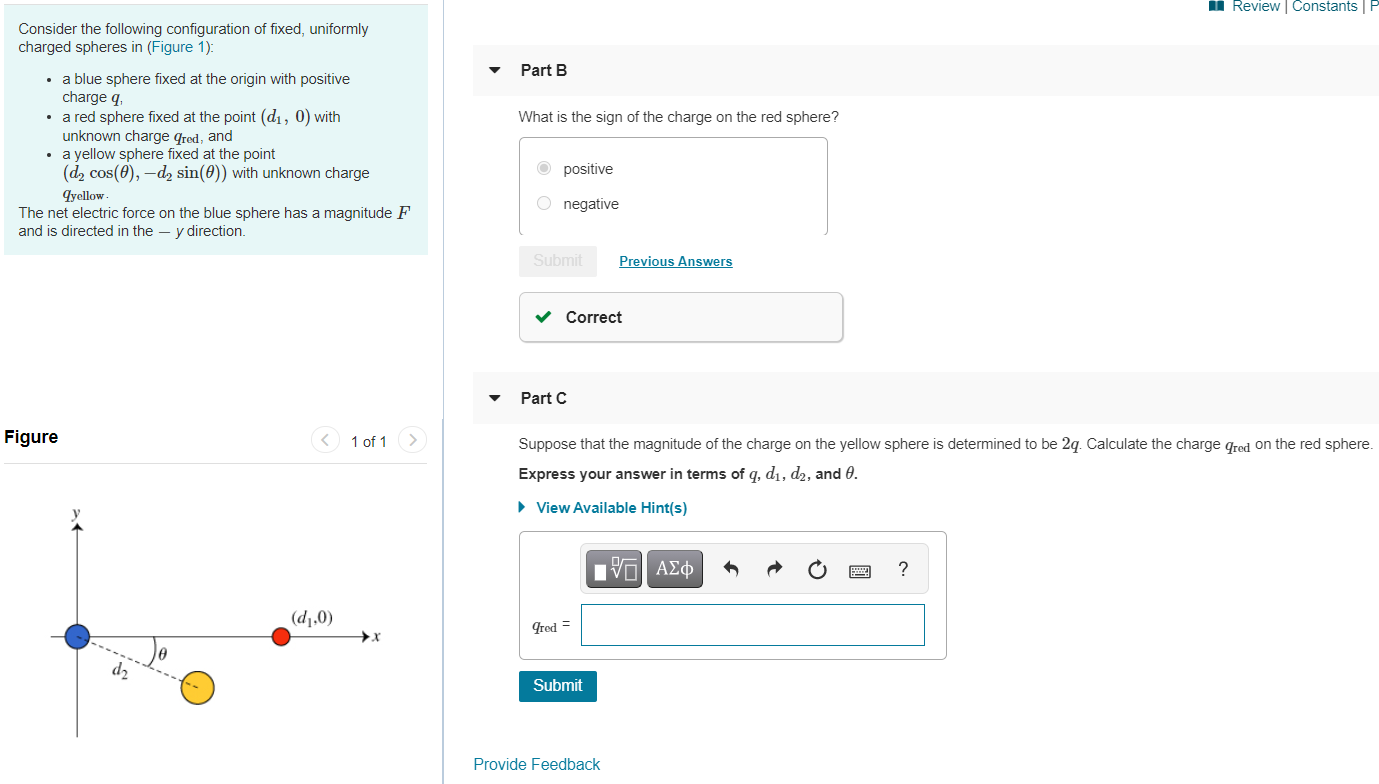# Question Solved1 Answer1 Review Constants Part B What is the sign of the charge on the red sphere? Consider the following configuration of fixed, uniformly charged spheres in (Figure 1): • a blue sphere fixed at the origin with positive charge a • a red sphere fixed at the point (di, 0) with unknown charge Cred, and • a yellow sphere fixed at the point (d, cos(Q), -d, sin(O)) with unknown charge qyellow The net electric force on the blue sphere has a magnitude F and is directed in the – y direction. o positive o negative Submit Previous Answers Correct Part C Figure < 1 of 1 Suppose that the magnitude of the charge on the yellow sphere is determined to be 24. Calculate the charge Gred on the red sphere. Express your answer in terms of q, di, d2, and 0. View Available Hint(s) ΙΡΙ ΑΣΦ ? (d,,0) Pred d2 Submit Provide FeedbackTranscribed Image Text: 1 Review Constants Part B What is the sign of the charge on the red sphere? Consider the following configuration of fixed, uniformly charged spheres in (Figure 1): • a blue sphere fixed at the origin with positive charge a • a red sphere fixed at the point (di, 0) with unknown charge Cred, and • a yellow sphere fixed at the point (d, cos(Q), -d, sin(O)) with unknown charge qyellow The net electric force on the blue sphere has a magnitude F and is directed in the – y direction. o positive o negative Submit Previous Answers Correct Part C Figure < 1 of 1 Suppose that the magnitude of the charge on the yellow sphere is determined to be 24. Calculate the charge Gred on the red sphere. Express your answer in terms of q, di, d2, and 0. View Available Hint(s) ΙΡΙ ΑΣΦ ? (d,,0) Pred d2 Submit Provide Feedback
More
Transcribed Image Text: 1 Review Constants Part B What is the sign of the charge on the red sphere? Consider the following configuration of fixed, uniformly charged spheres in (Figure 1): • a blue sphere fixed at the origin with positive charge a • a red sphere fixed at the point (di, 0) with unknown charge Cred, and • a yellow sphere fixed at the point (d, cos(Q), -d, sin(O)) with unknown charge qyellow The net electric force on the blue sphere has a magnitude F and is directed in the – y direction. o positive o negative Submit Previous Answers Correct Part C Figure < 1 of 1 Suppose that the magnitude of the charge on the yellow sphere is determined to be 24. Calculate the charge Gred on the red sphere. Express your answer in terms of q, di, d2, and 0. View Available Hint(s) ΙΡΙ ΑΣΦ ? (d,,0) Pred d2 Submit Provide Feedback